Our office will be closed from December 20, 2023 to January 02, 2024. Orders placed during this time will be processed starting on January 03, 2024.

# Understanding the basics of laser diode drivers

2018-10-16

In its most basic form, a laser driver is a current source built with a current-sense resistor and an operational amplifier. The operational amplifier measures the voltage across the sense resistor and controls its output in a feedback loop to maintain the resistor voltage as close as possible to the control voltage.

Since no current flows into the amplifier negative input, the laser current IL is equal to the control voltage VC divided by the sense resistor RS.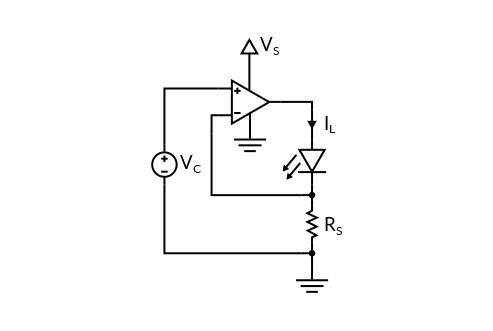The output stage of most opamps cannot supply more than a few tens of mA, it is thus common to replace it by a discrete transistor: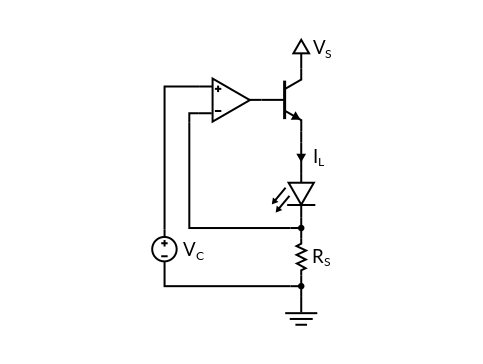### Compliance voltage

A laser driver can only regulate the current as long as the laser voltage stays within certain limits. The supply voltage VS is the sum of the sense resistor voltage VRs = RS x IL, the laser voltage VL and the transistor voltage VT.

The transistor can been seen as a variable resistor controlled by the opamp. When the laser voltage increases, the opamp tries to reduce the transistor resistance RT to maintain a constant current. At some point, the transistor resistance reaches its minimum value RTmin and the driver behaves as if the laser was supplied with VS, in series with RTmin and RS.

The compliance voltage is the maximum laser voltage at which the driver maintains current regulation. This voltage depends on the current and is usually specified at the maximum operating current of the driver.

### Noise analysis

At the input of the opamp, we can consider three voltage noise sources: the noise of the control voltage vC2, the input-referred noise of the op-amp vO2 and the thermal noise of the sense resistor vR2 = 4 kB T RS.

Let's consider a 100 mA laser driver made of a 10 Ω sense resistor, an opamp with 0.85 nV/√Hz input voltage noise and a noise-free control voltage. At room temperature, the thermal noise of the 10 Ω resistor is about 0.4 nV/√Hz. Since the two voltage noises are independent, they sum up to a power spectral density of (0.42+0.852)½ = 1.0 nV/√Hz. Dividing the result by 10 Ω, we obtain a current noise of 100 pA/√Hz.

It is possible to reduce the current noise by increasing the value of the sense resistor as shown in the graph below. At low resistor values, the thermal noise is negligible and current noise scales with the inverse of the resistance. Above about 50 Ω, the thermal noise starts to be preponderant and the current noise only scales with the inverse of the square root of the resistance.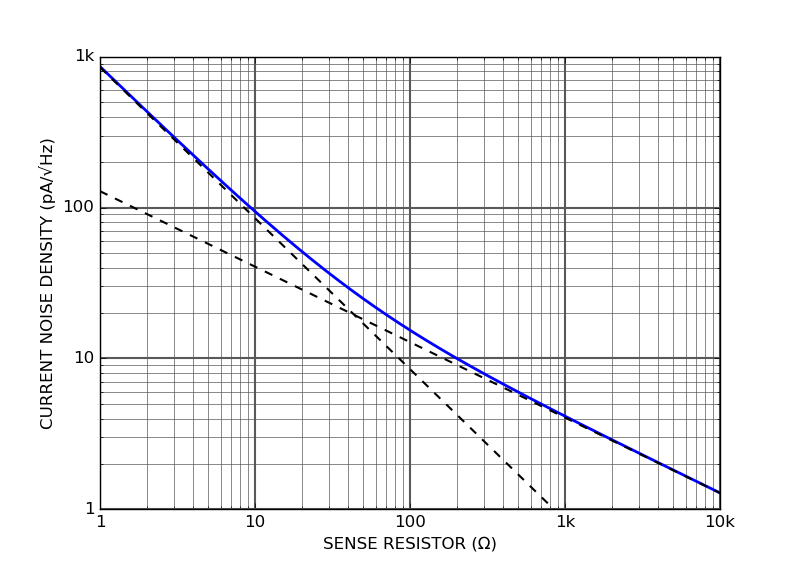The choice of the resistor value is a trade-off between current noise and power consumption.

### Modulating the laser current

Modulation can be performed in at least two ways, depending on the required modulation frequency. As long as the modulation frequency is smaller than the bandwidth of the feedback loop, the laser current can be modulated via the control voltage VC. This bandwidth is usually between a few kHz and a few MHz.

Above the driver's modulation bandwidth, laser current can be modulated with a bias-tee, as shown in the figure below: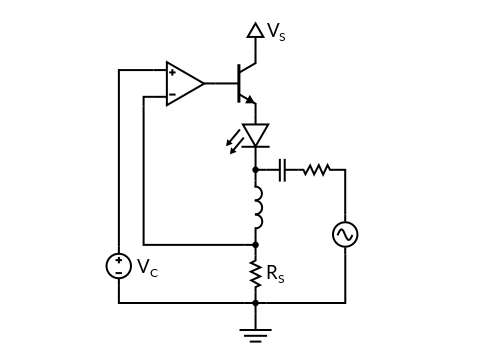The capacitor allows the AC modulation to pass through the laser while blocking the DC signal. The inductor, which isolates the driver from the AC modulation, must be small enough not to add too much phase within the driver's bandwidth.

### Grounding configurations

Some lasers diodes have their positive side (anode) or negative side (cathode) connected to the diode's metal case. If the metal case has to be connected to the ground, it is necessary to use an anode grounded or a cathode grounded laser driver, as shown in the figure below: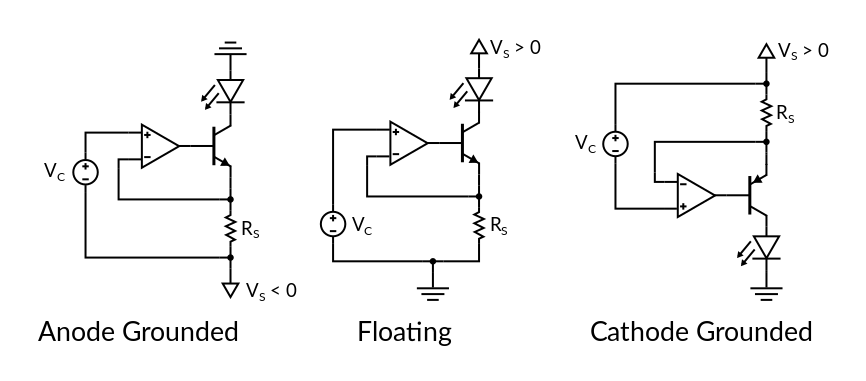Anode grounded drivers work from a negative supply while cathode grounded drivers work from a positive supply. In most situations, the diode's metal case can be electrically isolated from the ground so that a floating architecture can be used. In this architecture, the control electronics operate closer to the ground, which often leads to improved power efficiency.